# 1 Introduction

The aim of `cartography` is to obtain thematic maps with the visual quality of those build with a classical mapping or GIS software.

Users of the package could belong to one of two categories: cartographers willing to use R or R users willing to create maps. Therefore, its functions have to be intuitive to cartographers and ensure compatibility with common R workflows.

`cartography` uses `sf` or `sp` objects to produce `base` graphics. As most of the internals of the package relies on `sf` functionalities, the preferred format for spatial objects is `sf`.

# 2 Features

`cartography`’s functions can be classified in the following categories :

• Symbology
Each function focuses on a single cartographic representation (e.g. proportional symbols or choropleth representation) and displays it on a georeferenced plot. This solution allows to consider each representation as a layer and to overlay multiple representations on a same map.
Each function has two main arguments that are:

• `x`, a spatial object (preferably an `sf` object),
• `var`, the name of a variable to map.

`sp` objects are handled through the `spdf` argument if the variable is contained within the `Spatial*DataFrame` and through `spdf`, `spdfid`, `df`, `dfid` if the variable is in a separate `data.frame` that needs to be joined to the `Spatial*DataFrame`.

Many parameters are available to fine tune the cartographic representations. These parameters are the common ones found in GIS and automatic cartography tools (e.g. classification and color palettes used in choropleth maps, symbols sizes used in proportional symbols maps…).

• Transformations
A set of functions is dedicated to the creation or transformation of spatial objects (e.g. borders extraction, grid or links creation). These functions are provided to ease the creation of some more advanced maps that usually need geo-processing.

• Map Layout
Along with the cartographic functions, some other functions are dedicated to layout design (e.g. customizable scale bar, north arrow, title, sources or author information…).

• Color Palettes
16 original color palettes are shipped within the package. Those palettes can be customized and combined.

• Legends
Legends are displayed by default along cartographic layers but more parameters are available through `legend*()` functions.

• Classification
`getBreaks()` give access to most of the classification methods used for data binning.

# 3 Examples of thematic maps

## 3.1 OpenStreetMap Basemap and Proportional Symbols

`getTiles()` and `tilesLayer()` download and display OpenStreetMap tiles. Be careful to cite the source of the tiles appropriately.

`propSymbolsLayer()` displays symbols with areas proportional to a quantitative variable (stocks). Several symbols are available (circles, squares, bars). The `inches` argument is used to customize the symbols sizes.

``````library(sf)
library(cartography)
# path to the geopackage file embedded in cartography
path_to_gpkg <- system.file("gpkg/mtq.gpkg", package="cartography")
# import to an sf object
mtq <- st_read(dsn = path_to_gpkg, quiet = TRUE)
mtq.osm <- getTiles(
x = mtq,
type = "osm",
zoom = 11,
crop = TRUE
)
## Data and map tiles sources:
# plot osm tiles
tilesLayer(x = mtq.osm)
# plot municipalities (only borders are plotted)
plot(st_geometry(mtq), col = NA, border = "grey", add=TRUE)
# plot population
propSymbolsLayer(
x = mtq,
var = "POP",
inches = 0.4,
col = "brown4",
legend.pos = "topright",
legend.title.txt = "Total population"
)
# layout
layoutLayer(title = "Population Distribution in Martinique",
sources = "Sources: Insee and IGN, 2018\n© OpenStreetMap contributors.\nTiles style under CC BY-SA, www.openstreetmap.org/copyright.",
author = paste0("cartography ", packageVersion("cartography")),
frame = FALSE, north = FALSE, tabtitle = TRUE)
# north arrow
north(pos = "topleft")``````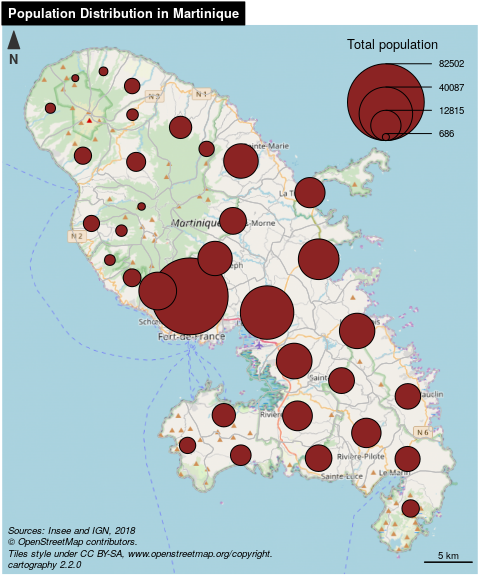## 3.2 Choropleth Map

In choropleth maps, areas are shaded according to the variation of a quantitative variable. They are used to represent ratios or indices.

`choroLayer()` displays choropleth maps . Arguments `nclass`, `method` and `breaks` allow to customize the variable classification. `getBreaks()` allow to classify outside of the function itself. Colors palettes are defined with `col` and a set of colors can be created with `carto.pal()` (see also `display.carto.all()`).

``````library(sf)
library(cartography)
# path to the geopackage file embedded in cartography
path_to_gpkg <- system.file("gpkg/mtq.gpkg", package="cartography")
# import to an sf object
mtq <- st_read(dsn = path_to_gpkg, quiet = TRUE)
# population density (inhab./km2) using sf::st_area()
mtq\$POPDENS <- 1e6 * mtq\$POP / st_area(mtq)
# plot municipalities (only the backgroung color is plotted)
plot(st_geometry(mtq), col = NA, border = NA, bg = "#aadaff")
# plot population density
choroLayer(
x = mtq,
var = "POPDENS",
method = "geom",
nclass=5,
col = carto.pal(pal1 = "sand.pal", n1 = 5),
border = "white",
lwd = 0.5,
legend.pos = "topright",
legend.title.txt = "Population Density\n(people per km2)",
)
# layout
layoutLayer(title = "Population Distribution in Martinique",
sources = "Sources: Insee and IGN, 2018",
author = paste0("cartography ", packageVersion("cartography")),
frame = FALSE, north = FALSE, tabtitle = TRUE, theme= "sand.pal")
# north arrow
north(pos = "topleft")``````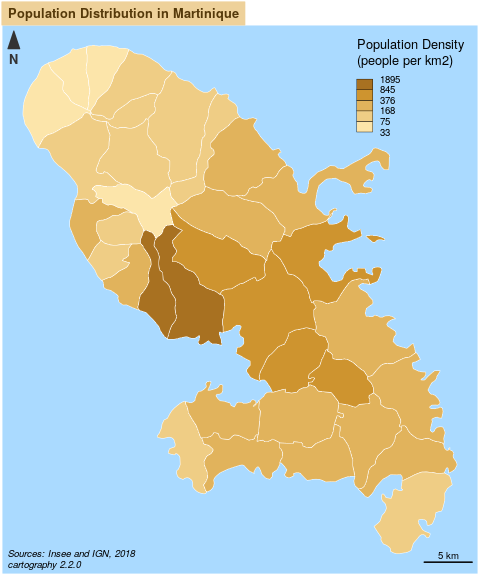## 3.3 Colored Pencil and Typologies Map

`getPencilLayer()` transforms POLYGONS or MULTIPOLYGONS in MULTILINESTRINGS. This function creates a layer that mimicks a pencil hand-drawing.

`typoLayer()` displays a typology map of a qualitative variable. `legend.values.order` is used to set the modalities order in the legend.

``````library(sf)
library(cartography)
# path to the geopackage file embedded in cartography
path_to_gpkg <- system.file("gpkg/mtq.gpkg", package="cartography")
# import to an sf object
mtq <- st_read(dsn = path_to_gpkg, quiet = TRUE)
# transform municipality multipolygons to (multi)linestrings
mtq_pencil <- getPencilLayer(
x = mtq,
size = 400,
lefthanded = F
)
# plot municipalities (only the backgroung color is plotted)
plot(st_geometry(mtq), col = "white", border = NA, bg = "lightblue1")
typoLayer(
x = mtq_pencil,
var="STATUS",
col = c("aquamarine4", "yellow3","wheat"),
lwd = .7,
legend.values.order = c("Prefecture",
"Sub-prefecture",
"Simple municipality"),
legend.pos = "topright",
legend.title.txt = "",
)
#  plot municipalities
plot(st_geometry(mtq), lwd = 0.5, border = "grey20", add = TRUE, lty = 3)
# labels for a few  municipalities
labelLayer(x = mtq[mtq\$STATUS != "Simple municipality",], txt = "LIBGEO",
cex = 0.9, halo = TRUE, r = 0.15)
# title, source, author
sources = "Sources: Insee and IGN, 2018",
author = paste0("cartography ", packageVersion("cartography")),
north = FALSE, tabtitle = TRUE, postitle = "right",
col = "white", coltitle = "black")
# north arrow
north(pos = "topleft")``````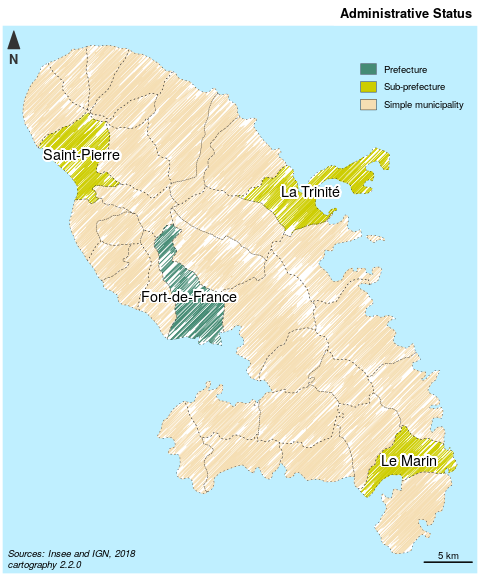## 3.4 Proportional Symbols and Choropleth Map

`propSymbolsChoroLayer()` creates a map of symbols that are proportional to values of a first variable and colored to reflect the classification of a second variable. A combination of `propSymbolsLayer()` and `choroLayer()` arguments is used.

``````library(sf)
library(cartography)
# path to the geopackage file embedded in cartography
path_to_gpkg <- system.file("gpkg/mtq.gpkg", package="cartography")
# import to an sf object
mtq <- st_read(dsn = path_to_gpkg, quiet = TRUE)
# Plot the municipalities
plot(st_geometry(mtq), col="darkseagreen3", border="darkseagreen4",
bg = "lightblue1", lwd = 0.5)
# Plot symbols with choropleth coloration
propSymbolsChoroLayer(
x = mtq,
var = "POP",
inches = 0.4,
border = "grey50",
lwd = 1,
legend.var.pos = "topright",
legend.var.title.txt = "Population",
var2 = "MED",
method = "equal",
nclass = 4,
col = carto.pal(pal1 = "sand.pal", n1 = 4),
legend.var2.values.rnd = -2,
legend.var2.pos = "left",
legend.var2.title.txt = "Median\nIncome\n(in euros)"
)
# layout
layoutLayer(title="Population & Wealth in Martinique, 2015",
author = "cartography 2.1.3",
sources = "Sources: Insee and IGN, 2018",
scale = 5, tabtitle = TRUE, frame = FALSE)
# north arrow
north(pos = "topleft")``````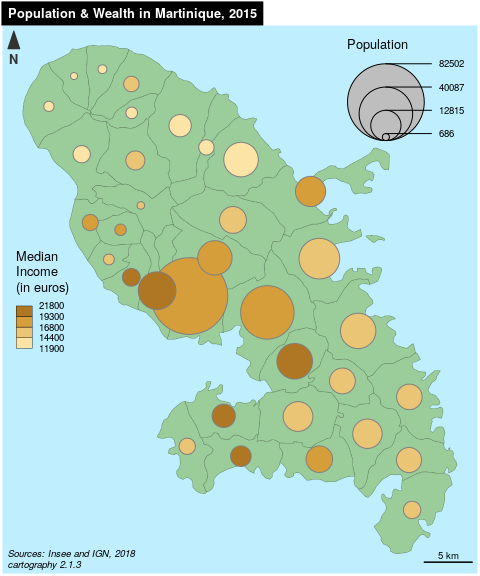## 3.5 Proportional Symbols and Typology Map

`propSymbolsTypoLayer()` creates a map of symbols that are proportional to values of a first variable and colored to reflect the modalities of a second qualitatice variable. A combination of `propSymbolsLayer()` and `typoLayer()` arguments is used.

``````library(sf)
library(cartography)
# path to the geopackage file embedded in cartography
path_to_gpkg <- system.file("gpkg/mtq.gpkg", package="cartography")
# import to an sf object
mtq <- st_read(dsn = path_to_gpkg, quiet = TRUE)
# Plot the municipalities
plot(st_geometry(mtq), col="#f2efe9", border="#b38e43", bg = "#aad3df",
lwd = 0.5)
# Plot symbols with choropleth coloration
propSymbolsTypoLayer(
x = mtq,
var = "POP",
inches = 0.5,
symbols = "square",
border = "white",
lwd = .5,
legend.var.pos = "topright",
legend.var.title.txt = "Population",
var2 = "STATUS",
legend.var2.values.order = c("Prefecture", "Sub-prefecture",
"Simple municipality"),
col = carto.pal(pal1 = "multi.pal", n1 = 3),
legend.var2.pos = c(693000, 1607000),
)
# layout
layoutLayer(title="Population Distribution in Martinique",
author = "cartography 2.1.3",
sources = "Sources: Insee and IGN, 2018",
scale = 5, tabtitle = TRUE, frame = FALSE)
# north arrow
north(pos = "topleft")``````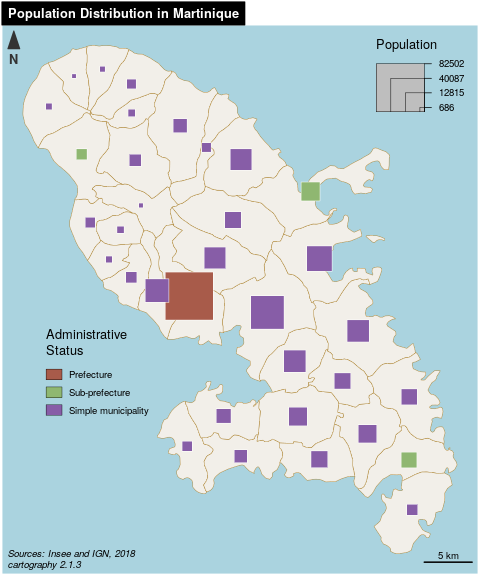## 3.6 Label Map

`labelLayer()` is dedicated to the display of labels on a map. The `overlap = FALSE` argument displays non overlapping labels.

``````library(sf)
library(cartography)
# path to the geopackage file embedded in cartography
path_to_gpkg <- system.file("gpkg/mtq.gpkg", package="cartography")
# import to an sf object
mtq <- st_read(dsn = path_to_gpkg, quiet = TRUE)
# plot municipalities
plot(st_geometry(mtq), col = "#e4e9de", border = "darkseagreen4",
bg = "lightblue1", lwd = 0.5)
# plot labels
labelLayer(
x = mtq,
txt = "LIBGEO",
col= "black",
cex = 0.7,
font = 4,
halo = TRUE,
bg = "white",
r = 0.1,
overlap = FALSE,
show.lines = FALSE
)
# map layout
layoutLayer(
title = "Municipalities of Martinique",
sources = "Sources: Insee and IGN, 2018",
author = paste0("cartography ", packageVersion("cartography")),
frame = FALSE,
north = TRUE,
tabtitle = TRUE,
theme = "taupe.pal"
) ``````